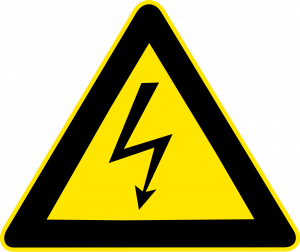Electrical circuits can be used for sending signals, storing information, or doing calculations, but their most common purpose by far is to manipulate energy. We know that light bulbs are rated in units of watts, i.e., how many joules per second of energy they can convert into heat and light, but how would this relate to the flow of charge as measured in amperes? By way of analogy, suppose your friend, who did not take physics, cannot find any job better than pitching bales of hay. The number of calories he burns per hour will certainly depend on how many bales he pitches per minute, but it will also be proportional to how much mechanical work he has to do on each bale. If his job is to toss them up into a hayloft, he will get tired a lot more quickly than someone who merely tips bales off a loading dock into trucks. In metric units,One-stop solution for all your homework needs. Get the job done.

✅ AI Essay Writer ✅ AI Detector ✅ Plagiarism checker ✅ Paraphraser

joulessecond=haybalessecond×jouleshaybale.

Similarly, the rate of energy transformation by a battery will not just depend on how many coulombs per second it pushes through a circuit, but also on how much mechanical work it has to do on each coulomb of charge:

joulessecond=coulombssecond×joulescoulomb

or

power=current×work per unit charge.

Units of joules per coulomb are abbreviated as volts, 1 V=1 J/C, named after the Italian physicist Alessandro Volta. Everyone knows that batteries are rated in units of volts, but the voltage concept is more general than that; it turns out that voltage is a property of every point in space. To gain more insight, let us think more carefully about what goes on in the battery and bulb circuit.

To do work on a charged particle, the battery apparently must be exerting forces on it. How does it do this? Well, the only thing that can exert an electrical force on a charged particle is another charged particle. It is as though the haybales were pushing and pulling each other into the hayloft! This is potentially a horribly complicated situation. Even if we knew how much excess positive or negative charge there was at every point in the circuit (which realistically we do not) we would have to calculate zillions of forces using Coulomb’s law, perform all the vector additions, and finally calculate how much work was being done on the charges as they moved along. To make things even more scary, there is more than one type of charged particle that moves: electrons are what move in the wires and the bulb’s filament, but ions are the moving charge carriers inside the battery. Luckily, there are two ways we can simplify things:

The situation is unchanging. Unlike the imaginary setup in which we attempted to light a bulb using a rubber rod and a piece of fur, this circuit maintains itself in a steady state (after perhaps a microsecond-long period of settling down after the circuit is first assembled). The current is steady, and as charge flows out of any area of the circuit, it is replaced by the same amount of charge flowing in. The amount of excess positive or negative charge in any part of the circuit therefore stays constant. Similarly, when we watch a river flowing, the water goes by but the river does not disappear.

Force depends only on position. Since the charge distribution is not changing, the total electrical force on a charged particle depends only on its own charge and on its location. If another charged particle of the same type visits the same location later on, it will feel exactly the same force.

The second observation tells us that there is nothing all that different about the experience of one charged particle as compared to another. If we single out one particle to pay attention to, and figure out the amount of work done on it by electrical forces as it goes from point A to point B along a certain path, then this is the same amount of work that will be done on any other charged particles of the same type as it follows the same path. For the sake of visualization, let us think about the path that starts at one terminal of the battery, goes through the light bulb’s filament, and ends at the other terminal. When an object experiences a force that depends only on its position (and when certain other, technical conditions are satisfied), we can define an electrical energy associated with the position of that object—the amount of work done on the particle by electrical forces as it moves from A to B equals the drop in electrical energy between A and B. This electrical energy is what is being converted into other forms of energy such as heat and light. We therefore define voltage in general as electrical energy per unit charge.

The difference in voltage between two points in space is defined as

ΔV=ΔPEelec/q,

where ΔPEelecΔPEelec is the change in the electrical energy of a particle with charge qq as it moves from the initial point to the final point.

The amount of power dissipated (i.e., rate at which energy is transformed by the flow of electricity) is then given by the equation

P=IΔV.